## Algebra

Showing posts with label complete the square. Show all posts
Showing posts with label complete the square. Show all posts

### Hyperbolas

An hyperbola is the set of all points whose distances to two fixed points subtract to the same constant. In this section, we will focus on the equation of hyperbolas.The axis that contains the vertices is called the transverse axis and the axis that does not contain the vertices is called the conjugate axis. Also notice that the shape is very different than that of a parabola, hyperbolas are asymptotic to the following lines:We will make use of these asymptotes to sketch the graphs of hyperbolas.[ Interactive: Hyperbolas ]
To easily graph the asymptotes of a hyperbola use the following process.

Step 1: Identify the center (h, k) from standard form.
Step 2: Plot points a units left and right from center.
Step 3: Plot points b units up and down from center.
Step 4: Draw the rectangle defined by these points.
Step 5: The lines though the corners of this rectangle are the asymptotes!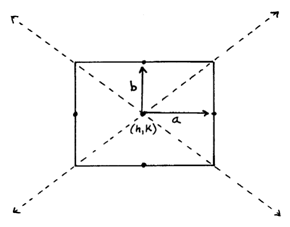Finally use the equation to determine if the parabola is vertical or horizontal.We knew to draw the above hyperbola opening to the left and right because the x^2 term was positive.  If the x^2 term is negative then the hyperbola opens up and down.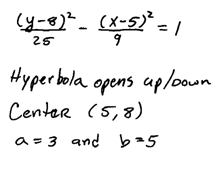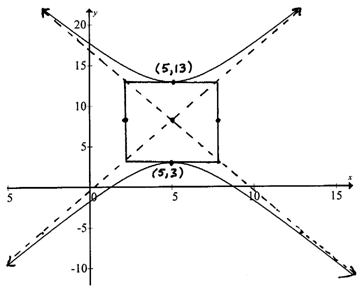Compare this hyperbola to the previous and note the difference in standard form. The rectangle and asymptotes are not actually part of the graph. We use these to obtain a more accurate sketch.  When graphed on a graphing utility the result looks like this.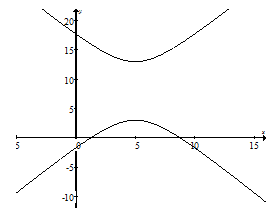Graph the hyperbola and give the equations of the asymptotes.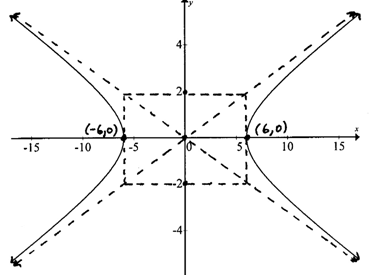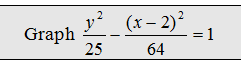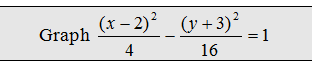The next examples require us to complete the square to obtain standard form.  Remember to factor the leading coefficient out of each variable grouping before using (B/2)^2 to complete the square.

Graph the hyperbola, x- and y- intercepts, and give the equations of the asymptotes.When we completed the square, notice that we added 64 and subtracted 225 to balance the equation.  It may appear, at first glance, that we added 16 and 25 to the left side. But in reality, after we distribute the 4 and -9 we added and subtracted larger values.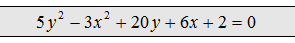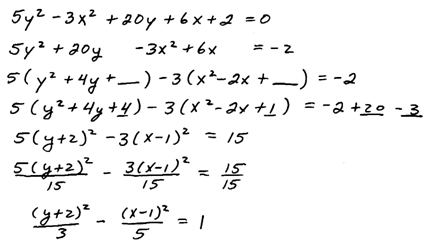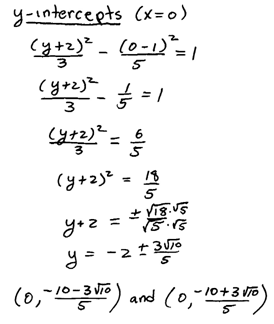When the transverse axis is vertical be careful with the center.  A common error is to use (-2, 1) for the center in the above example because the y variable comes first and we are used to reading these from left to right.  This would be incorrect so take care to use the correct h and k.

Example: Find the equation of the hyperbola centered at (-3, 1) where a = 2, b = 3, and with a vertical transverse axis.  Graph it.Example: Find the equation of the hyperbola with vertices (3,0) and (-3,0), horizontal transverse axis, and conjugate axis of length 4 units.Example: Find the equation of the hyperbola with center (2, -5), vertical transverse axis measuring 10 units, and conjugate axis measuring 6 units.YouTube Videos: Click problem to see it worked out.

---

### Circles

A circle is the set of points equidistant from a fixed point called the center. The distance from the center to any point is called the radius.  Recall the following formulas from Geometry: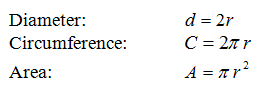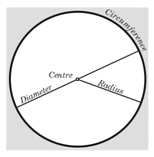The circumference can be thought of as the perimeter of the circle.  In this section, we will be interested in the equations that describe circles.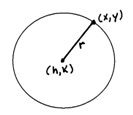From the equation of a circle in standard form we can see that a circle is completely determined by its center and radius.  In addition, notice that it is not a function because it fails the vertical line test.
[ InteractiveCircles ]

Given the equation of a circle in standard form, you can graph it using the following steps:
1. Plot the center (hk).
2. Use the radius r to plot four points up, down, left and right of the center.
3. Connect the points and label them.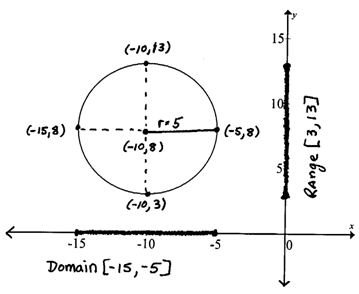Even though a circle is not a function, it is a relation and we could still find the domain and range.  In addition, we will be asked to find the x- and y-intercepts.  This particular example does not have any but the technique to find them is the same method used throughout this study guide.

To find y-intercepts set x = 0 and solve for y.
To find x-intercepts set y = 0 and solve for x.

Graph the circles and label the x- and y-intercepts.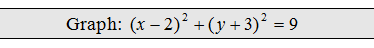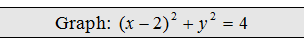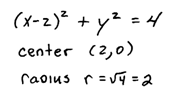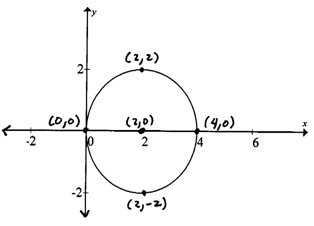Graphing circles in standard form is just a matter of identifying the center and the radius.  The difficulty comes when the circle is not given in standard form.  In this case, when given general form, we will complete the square twice as illustrated below.
1. Group all terms with variable x and group all terms with variable y.
2. Complete the square for each grouping.
3. Be sure to add (b/2)^2 to both sides of the equation.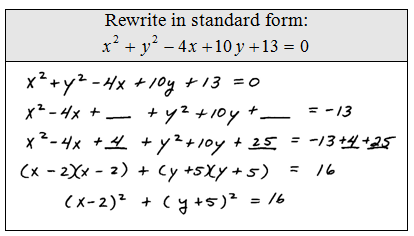Rewrite the circles in standard form and identify the center.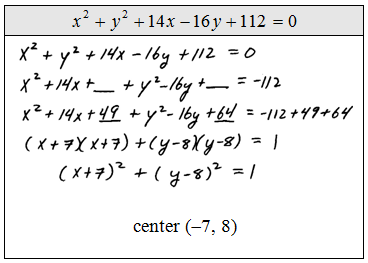Find the area of the following circles.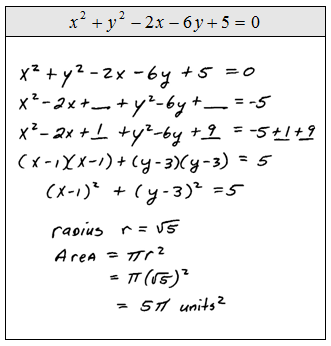Graph and find the x- and y-intercepts, area, and circumference.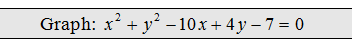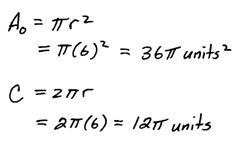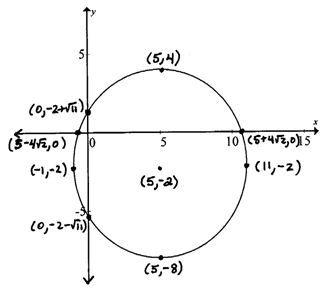Given the center and radius, find the equation of the circle.